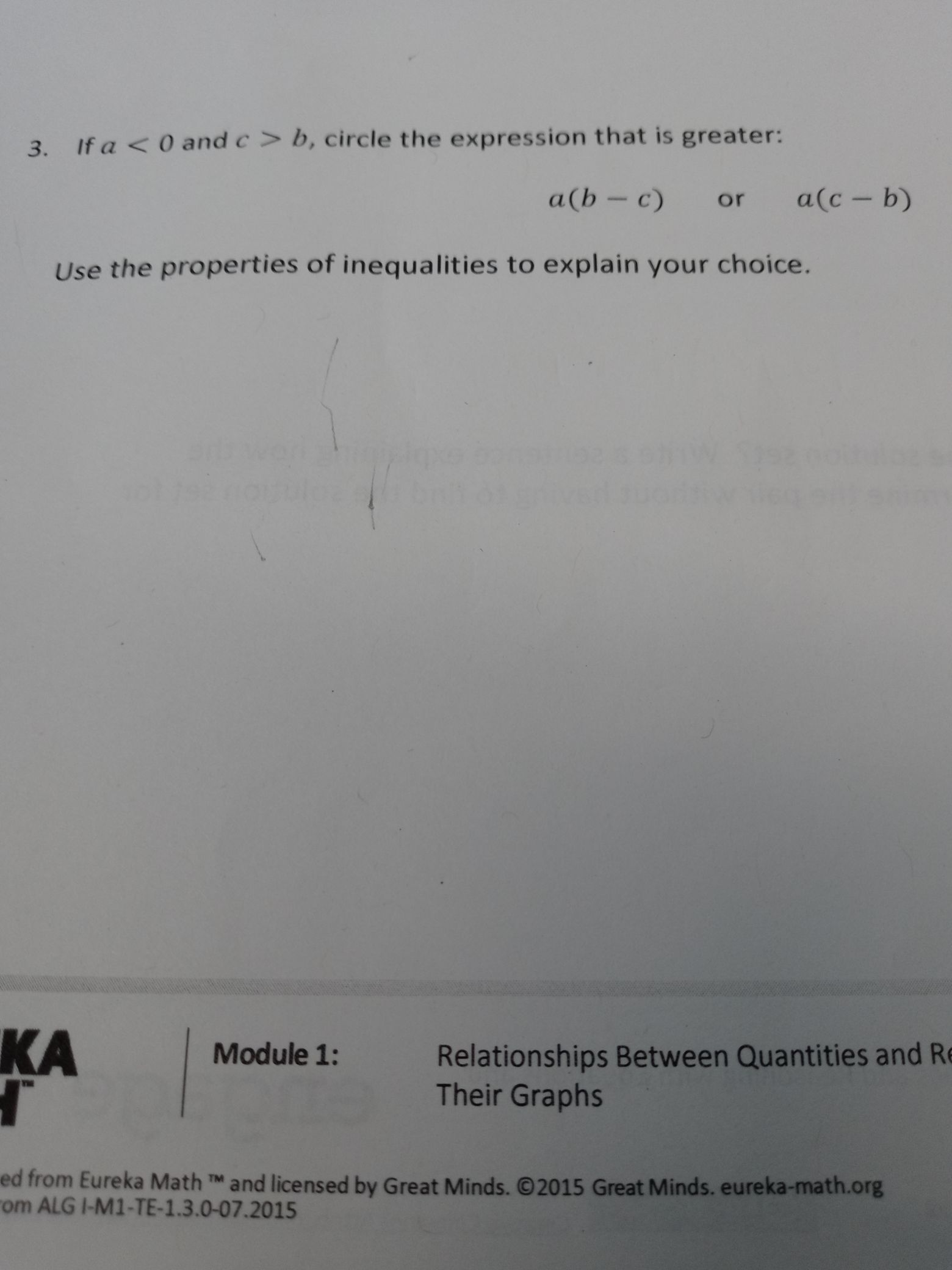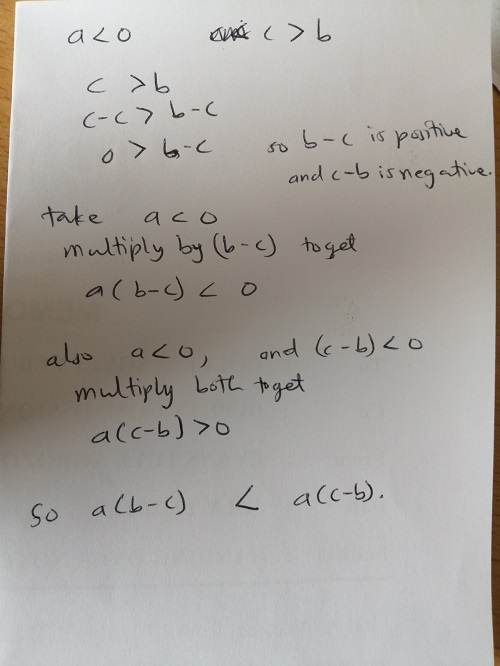### ¿Todavía tienes preguntas de matemáticas?

Pregunte a nuestros tutores expertos
Algebra
Pregunta3. If $$a < 0$$ and $$c > b$$ , circle the expression that is greater: $$a ( b - c )$$ or $$a ( c - b )$$ Use the properties of inequalities to explain your choice.